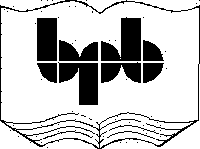This chapter traverses to the innards of the executable file in order to fathom the concept of metadata.

a.cs

using System;

using System.IO;

public class zzz

{

public static void Main ()

{

zzz a = new zzz();

a.abc();

}

public void abc()

{

FileStream s = new FileStream("C:\\mdata\\b.exe",FileMode.Open);

s.Seek(128+4+20+208, SeekOrigin.Begin);

int rva,size;

int where = rva%0x2000 + 512;

s.Seek(where + 4 + 4, SeekOrigin.Begin);

where = rva%0x2000 + 512;

s.Seek(where, SeekOrigin.Begin);

byte a,b,c,d;

Console.WriteLine("{0}{1}{2}{3}" , (char)a , (char)b , (char)c , (char)d);

Console.WriteLine("Major Version {0}", major);

Console.WriteLine("Minor Version {0}", minor);

Console.WriteLine("Reserved {0}", reserved );

Console.WriteLine("Length of string {0}", len);

for ( int i = 1 ; i <= len ; i++)

{

Console.Write("{0}",(char) e);

}

Console.WriteLine();

int leftover = len % 4;

Console.WriteLine("Four Byte boundary {0}" , leftover );

for ( int  i = 1 ; i <= leftover ; i++)

s.Seek(1 , SeekOrigin.Current);

Console.WriteLine("Flags {0}", flags);

}

}

Output

BSJB

Major Version 1

Minor Version 1

Reserved 0

Length of string 12

v1.0.3328

Four Byte boundary 0

Flags 0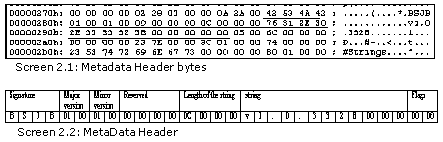The program sets sail by proceeding directly to the 15 Data Directory entry, from where the CLR header commences. After extracting the address in the file, we travel to that location. At this location, 8 bytes from the start is posted another Data Directory entry for the MetaData. Using the earlier approach, we jump to the beginning of the MetaData on disk.

The first 4 bytes of the MetaData header contain another Magic Number BSJB, which again comprises of the initials of the four guys who built the MetaData standard. The J stands for Jim Hess. The MetaData structures, as you would shortly witness, is a fine work of art.

Then, we encounter the Major and Minor versions of the MetaData, which are 1 and 0, respectively. The documentation lucidly states that these values can be eschewed for the time being. Close on its heel is an int, which is marked as reserved. Next in sequence is yet another int, which specifies the length of the string that ensues.

The string length is specified as 12, which signifies the fact that the next 12 bytes contain a string value. On displaying the 12 bytes, a value of v1.0.3328 becomes apparent.

At the command prompt, specify the following command:

Output

c:\mdata>csc

Microsoft (R) Visual C# .NET Compiler version 7.00.9372.1

for Microsoft (R) .NET Framework version 1.0.3328

Notice that the .Net framework version corresponds to the string value. In order to arrive at the next byte, we have to surmount the drawback faced by the current 32-bit machines. As per the specifications of such machines, everything is aligned on a four-byte boundary.

Therefore, after dividing the length by 4, the remainder that is obtained, is the number of bytes by which to move ahead. In the world of metadata, alignment on a four-byte boundary still prevails. In our case, since the string length is 12, no padding is essential. Lastly, we come across the flags field having a value of 0.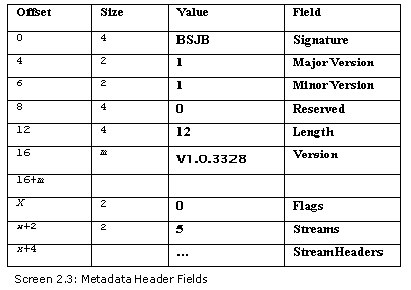From the above diagram, it would appear as though the last two fields have been omitted by us. However, that is not the case. We realized that a separate program was crucial to cater to the stream fields. Thus, we have incorporated the program given below, which describes the stream entity.

a.cs

using System;

using System.IO;

public class zzz

{

public static void Main ()

{

zzz a = new zzz();

a.abc();

}

public void abc()

{

FileStream s = new FileStream("C:\\mdata\\b.exe",FileMode.Open);

s.Seek(128+4+20+208, SeekOrigin.Begin);

int rva,size;

int clihdr= rva%0x2000 + 512;

Console.WriteLine("clihdr on disk: " +clihdr);

s.Seek(clihdr + 4 + 4, SeekOrigin.Begin);

clihdr = rva%0x2000 + 512;

s.Seek(clihdr, SeekOrigin.Begin);

s.Seek(4 + 2 + 2 + 4 + 4 + 12 + 2, SeekOrigin.Current);

Console.WriteLine("No of Streams {0}", streams);

int [] offset = new int[streams];

int [] ssize  = new int[streams];

int i = 0;

for ( i = 0 ; i < streams ; i++)

{

Console.Write("Offset {0} Size {1} ", offset[i], ssize[i]);

while ( true )

{

if ( b == 0)

break;

Console.Write("{0}", (char) b);

}

Console.WriteLine();

while ( true )

{

if ( s.Position % 4 == 0 )

break;

if ( b != 0)

{

s.Seek(-1, SeekOrigin.Current);

break;

}

}

}

}

}

Output

clihdr on disk: 520

Start of Metadata on disk  : 636

No of Streams 5

Offset 108 Size 208 #~

Offset 316 Size 116 #Strings

Offset 432 Size 12 #US

Offset 444 Size 16 #GUID

Offset 460 Size 36 #Blob

This program is a progression from the previous one. The starting position of the metadata in the file is stored in a variable, aptly named as startofmetadata. This variable is put to extensive use in the next program. The values in the variables are displayed merely for the sake of convenience and verification.

After arriving at the metadata in the file, the next 30 bytes of the Metadata header are traversed. Their details have already been displayed in the previous program.

The next two bytes in the sequence contain the count of the number of streams that are present in the MetaData. There will always be a maximum of 5 streams.

Everything in the MetaData world is stored in streams. This is the basic entity that is to be dealt with, while retrieving any information on the metadata. Following the field that contains the number of streams, are the stream headers.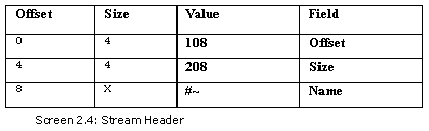The stream header adheres to a specific pattern. Since there are a total of 5 streams, there subsist 5 stream headers. A stream header comprises of an offset, the size and the stream name. The offset is with respect to the metadata position. The size is always in multiples of 4, in keeping with the alignment problem, and it indicates the size of the stream data on disk. Finally, there exists the name, which is null terminated. However, bytes are padded to cater to any complexities in alignment.

Two integer arrays are created for each stream, in order to store the offset and the size.

Contingent to the value stored in the streams, an array is created to store the offset and the size. 4 bytes each for the offset and for the size are read into the respective arrays. Thereafter, every byte is read till a null or a 0 value is encountered. Simultaneously, each byte is displayed in the ASCII format. The alignment of 4 has also been taken into account, after successfully reading the stream.

The downside of the MetaData world is that its specifications have been designed for efficiency, and not for straightforward comprehension. Therefore, in order to maintain efficiency, the string is padded with zeroes.

Before we narrow down further to display the data that is stored at these string offsets, we need to discern the concept of bitwise shift operators.

a.cs

using System;

public class zzz

{

public static void Main ()

{

Console.WriteLine( 3 >> 1);

Console.WriteLine( 8 >> 2);

Console.WriteLine( 9 >> 3);

}

}

Output

1

2

1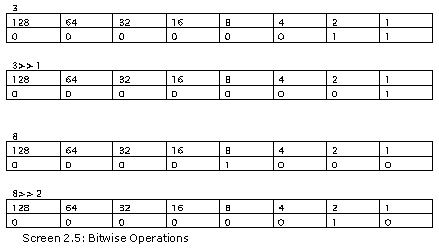The number 3 has the first two bits on. The >> sign shifts the bits to the right. Thus, using >> 1, all the bits are moved one position to the right, resulting in the first bit falling off the edge, and the second bit becoming the first bit, and so on. The bit on the extreme left is assigned a new value of zero. Thus, the final answer is 1.

In the second case, the number 8 has the fourth bit on. By shifting twice to the right, all the bits get shifted by two, resulting in the new value of 2. The same procedure is repeated in the third case also, where the fourth and the first bits are on. The first bit falls off and the fourth bit becomes the first bit.

The inverse of this is the left shift operator, where the operator that is used is << . Here, the rightmost bit falls off during left shifting, and a value of 0 is assigned to the newly introduced rightmost bit.

The right shifting action results in the division operation. Thus, right shifting by 2 results in a division by 4, and so on so forth. This is in contrast to left shifting, which results in multiplication.

a.cs

using System;

using System.IO;

public class zzz

{

public static void Main ()

{

zzz a = new zzz();

a.abc();

}

int [] offset;

int [] ssize ;

byte [] strings;

byte [] us;

byte [] guid;

byte [] blob;

long valid, sorted;

int nooftables;

byte [][] names;

public void abc()

{

FileStream s = new FileStream("C:\\mdata\\b.exe",FileMode.Open);

s.Seek(360, SeekOrigin.Begin);

int rva,size;

int where = rva%0x2000 + 512;

s.Seek(where + 4 + 4, SeekOrigin.Begin);

where = rva%0x2000 + 512;

s.Seek(where, SeekOrigin.Begin);

s.Seek(4 + 2 + 2 + 4 + 4 + 12 + 2, SeekOrigin.Current);

offset = new int;

ssize  = new int;

names = new byte[];

names = new byte;

names = new byte;

names = new byte;

names = new byte;

names = new byte;

int i = 0; int j ;

for ( i = 0 ; i < streams ; i++)

{

j = 0;

byte bb ;

while ( true )

{

if ( bb == 0)

break;

names[i][j] = bb;

j++;

}

names[i][j] = bb;

while ( true )

{

if ( s.Position % 4 == 0 )

break;

if ( b != 0)

{

s.Seek(-1, SeekOrigin.Current);

break;

}

}

}

for ( i = 0 ; i < streams ; i++)

{

Console.Write("Offset {0} Size {1} " , offset[i],ssize[i] );

j = 0;

while ( true)

{

if ( names[i][j] == 0)

break;

Console.Write("{0}", (char)names[i][j]);

j++;

}

Console.WriteLine();

}

for ( i = 0 ; i < streams ; i++)

{

if ( names[i] == '~' )

{

for ( int k = 0 ; k < ssize[i] ; k ++)

}

if ( names[i] == 'S' )

{

strings = new byte[ssize[i]];

for ( int k = 0 ; k < ssize[i] ; k ++)

}

if ( names[i] == 'U' )

{

us = new byte[ssize[i]];

for ( int k = 0 ; k < ssize[i] ; k ++)

}

if ( names[i] == 'G' )

{

guid = new byte[ssize[i]];

for ( int k = 0 ; k < ssize[i] ; k ++)

}

if ( names[i] == 'B' )

{

blob = new byte[ssize[i]];

for ( int k = 0 ; k < ssize[i] ; k ++)

}

}

Console.WriteLine("Reserved {0}" , reserved);

Console.WriteLine("Tables Bit Vector {0}", valid.ToString("X"));

nooftables = 0;

for ( int k = 0 ; k <= 63 ; k++)

{

nooftables += (int)(valid >> k ) & 1;

}

Console.WriteLine("No of Tables {0}", nooftables );

Console.WriteLine("Sorted Tables Bit Vector {0}", sorted.ToString("X"));

}

}

Output

Offset 108 Size 208 #~

Offset 316 Size 116 #Strings

Offset 432 Size 12 #US

Offset 444 Size 16 #GUID

Offset 460 Size 36 #Blob

Reserved 0

Major Table Verison 1

Minor Table Verison 0

Heap Sizes 0

Reserved 1

Tables Bit Vector 900001447

No of Tables 8

Sorted Tables Bit Vector 2003301FA00

The above program launches the journey to comprehending the internals of metadata. After positioning the file pointer at the metadata header, we progress onto storing the names of the streams in an array. In the earlier program, we had merely displayed every byte from the name field.

To store the names, a large array of arrays is created, wherein each array holds a string. Since there are 5 streams, 5 sub-arrays are created. Besides this, the sub-array is assigned a size of 10, since it is our perception that not more than 10 stream names will be present.

Like earlier, in the 'for' loop, the offset and the size are read for each stream, and they are placed at their respective array locations. The variable j is used to index the inner array, and the variable i is used for indexing the outer one. On quitting out of the first 'while' loop, the name array is null-terminated merely to facilitate printing. Thus, the first enhancement over the previous program is that, all the stream names have been placed in an array.

In order to authenticate our action, the size, the offset and the name of each stream are printed. The names array is displayed using a 'while' loop, which terminates when it encounters a zero value. This explains the first half of the program.

The second half of the program deals with the data of the five streams. We have chosen to store the data of the five streams in five separate arrays. Not only does this accelerate the execution process of this program, but it also makes it easier to understand. The rules that apply to reading data from one stream into an array, remain the same for reading data from all the other streams.

Here, instance variables are created for the arrays, thereby permitting access to all the functions. The five instance variables are metadata, ~,strings, us, guid and blob. Then, using a 'for' loop that iterates five times, five 'if' statements are incorporated. The second byte of each stream name is checked for a specific character. The first byte is ignored since all stream names store a # character in the first byte. We could have compared the whole string though, but at the moment, we intend to make life easier and unexacting for you. Hence, we have adopted this approach.

If the second byte is ~, it is indicative of the fact that the stream stores the details or tables of metadata. We would be explaining this in a short while. The corresponding size array stores the size of the stream, which determines the size of the array. The corresponding offset array has a value, which points to the beginning of the stream data, as an offset from the start of the metadata header. The starting position of the metadata header is stored in the variable startofmetadata.

So, the file pointer is initially positioned at the start of this streams data, and then re-positioned at the offset variable. Now that we are positioned at the start of the stream data, we use a 'for' loop to populate the metadata array.

In place of bringing into play the ReadByte function that reads a single byte at a time, we could have used the ReadBytes function instead. The ReadBytes function reads a certain number of bytes from the current stream into a byte array, and thereon, veers the current file pointer position ahead by those many bytes. Wielding the same mechanism, the other four arrays are also filled up. As an outcome of this, five arrays are populated with data from the streams that constitute the metadata. The most imperative of all streams is the stream #~. So, let us untangle its format in detail.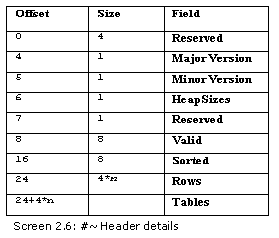The data for the #~ stream begins with four bytes that are reserved. Hence, they all have zero values.

We have employed the methods of the BinaryReader class and the BitConverter class. While the BinaryReader class reads multiple bytes at a time, the BitConvertor class converts the extracted bytes into a suitable type. The static method of ToInt32 in the BitConverter class takes two parameters, viz. the byte array and an offset. It picks up 32 bits from the offset in the array and converts them into a 32-bit or a 4-byte integer value.

The parameters supplied to this function are the array metadata and the offset of 0.

The value of 0 indicates that the function must read the first four bytes. In the case of an array, the counting starts from 0 and not from 1. The documentation states that this reserved field should always be 0.

This is followed by two bytes that contain the major and minor version numbers of the tables schema. As of now, the major version is 1 and the minor version is 0. Since they are straightforward bytes, we read them off the array itself. There is no necessity of implementing any method from the BitConverter class.

The next byte deals with heap sizes, which would be discussed shortly. The byte that follows the heap size is reserved with a value of 1. Following the reserved byte, the next eight bytes or long, embody a count of the number of tables that are present.

By now, you may have got thoroughly peeved at encountering the term 'table' every so often, without an explanation appended with it. Folks, sulk no more. We serve-up the explanation and unravel its mystery for you right away!

All metadata is stored internally in the form of tables. There are about 43 varied table types in the realm of metadata. All classes or types are stored in one table, while all the methods are stored in another table, and so on. Each table is assigned a bit in the table field. Contingent upon the status of this bit field, the presence of a particular table can be ascertained. Further, the quantum of tables present in a file, depends upon the number of bits that are switched on.

As of now, the largest bit for a table is 0x2b, i.e. 43. However, while some are never used, some are just incapable of storing any information.

Before we explore the entire gamut of tables, let us initially count the number of tables present in the file. A 'for' loop is used towards this end, which repeats the code 64 times. Thus, the variable k is assigned values ranging from 0 to 63. All the bits are right shifted k times, and then, a bitwise AND operation is performed with a value of 1.

We offer to elucidate the following statement once again in greater depth:

nooftables += (int)(valid >> k ) & 1;

The value of k is 0 on the first iteration of the 'for' loop. Therefore, the statement evaluates to valid >> 0. Right shifting by 0 prompts no change whatsoever in the bits in the field. The AND operation with 1 will now check if the rightmost bit contains the value of 1 or not. The final result would be either 1 or 0. So, either 1 or 0 gets added to the count variable of nooftables.

In the second iteration of the loop, when the value of the variable k is 1, the statement evaluates to: nooftables += (int)(valid >> 1 ) & 1;

Thus, all the bits in the field are pushed to the right by 1. As a consequence of this, the first bit gets discarded and the second bit becomes the first bit. If this bit is ON, the result obtained is 1, and the nooftables variable is incremented by 1.

In the next round, the statement becomes:

nooftables += (int)(valid >> 2 ) & 1;

Thus, in this manner, every bit in the valid field is validated to determine if it contains a value of 1 or not.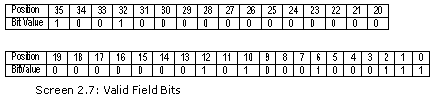The valid field is a bit vector, which has 8 bits on. Thus, 8 tables are finally created for the smallest exe file. The nooftables variable corroborates this value. The table field is followed by a long called the 'sorted tables bit vector'. We shall be attending to this field in a short while.

a.cs

using System;

using System.IO;

public class zzz

{

public static void Main ()

{

zzz a = new zzz();

a.abc();

}

string [] tablenames;

int tableoffset ;

int [] rows;

int [] offset;

int [] ssize ;

byte [] strings;

byte [] us;

byte [] guid;

byte [] blob;

long valid, sorted;

int nooftables;

byte [][] names;

public void abc()

{

FileStream s = new FileStream("C:\\mdata\\b.exe",FileMode.Open);

s.Seek(360, SeekOrigin.Begin);

int rva,size;

int where = rva%0x2000 + 512;

s.Seek(where + 4 + 4, SeekOrigin.Begin);

where = rva%0x2000 + 512;

s.Seek(where, SeekOrigin.Begin);

s.Seek(4 + 2 + 2 + 4 + 4 + 12 + 2, SeekOrigin.Current);

offset = new int;

ssize  = new int;

names = new byte[];

names = new byte;

names = new byte;

names = new byte;

names = new byte;

names = new byte;

int i = 0; int j ;

for ( i = 0 ; i < streams ; i++)

{

j = 0;

byte bb ;

while ( true )

{

if ( bb == 0)

break;

names[i][j] = bb;

j++;

}

names[i][j] = bb;

while ( true )

{

if ( s.Position % 4 == 0 )

break;

if ( b != 0)

{

s.Seek(-1, SeekOrigin.Current);

break;

}

}

}

for ( i = 0 ; i < streams ; i++)

{

if ( names[i] == '~' )

{

for ( int k = 0 ; k < ssize[i] ; k ++)

}

if ( names[i] == 'S' )

{

strings = new byte[ssize[i]];

for ( int k = 0 ; k < ssize[i] ; k ++)

}

if ( names[i] == 'U' )

{

us = new byte[ssize[i]];

for ( int k = 0 ; k < ssize[i] ; k ++)

}

if ( names[i] == 'G' )

{

guid = new byte[ssize[i]];

for ( int k = 0 ; k < ssize[i] ; k ++)

}

if ( names[i] == 'B' )

{

blob = new byte[ssize[i]];

for ( int k = 0 ; k < ssize[i] ; k ++)

}

}

nooftables = 0;

tableoffset = 24;

rows = new int;

Array.Clear (rows, 0, rows.Length);

for ( int k = 0 ; k <= 63 ; k++)

{

int tablepresent = (int)(valid >> k ) & 1;

if ( tablepresent == 1)

{

tableoffset += 4;

}

}

tablenames = new String[]{"Module" , "TypeRef" ,            "TypeDef" ,"FieldPtr","Field", "MethodPtr","Method","ParamPtr" , "Param", "InterfaceImpl", "MemberRef", "Constant", "CustomAttribute", "FieldMarshal", "DeclSecurity", "ClassLayout", "FieldLayout", "StandAloneSig" , "EventMap","EventPtr", "Event", "PropertyMap", "PropertyPtr", "Properties","MethodSemantics","

MethodImpl","ModuleRef","TypeSpec","ImplMap","FieldRVA","ENCLo

g","ENCMap","Assembly","AssemblyProcessor","AssemblyOS",

"AssemblyRef","AssemblyRefProcessor","AssemblyRefOS",

"File","ExportedType","ManifestResource","NestedClass",

"TypeTyPar","MethodTyPar"};

for ( int k = 0 ; k <= 63 ; k++)

{

if ( rows[k] != 0 )

Console.WriteLine("Table {0} Rows {1} ",tablenames[k] , rows[k]);

}

Console.WriteLine("Actual Tables start at {0}" , tableoffset);

}

}

Output

Table Module Rows 1

Table TypeRef Rows 3

Table TypeDef Rows 2

Table Method Rows 2

Table MemberRef Rows 3

Table CustomAttribute Rows 1

Table Assembly Rows 1

Table AssemblyRef Rows 1

Actual Tables start at 56

Now that we have established the fact that there are 8 tables in the file, the next task is to ascertain the table type and the number of rows contained in each. Most of the code above remains unaltered.

The tableoffset variable is set to 24, thereby circumventing the initial header members.

A 'for' loop is implemented as before, with the value of k ranging from 0 to 63. Earlier, the result of the right shift operation (which could either be the value of 1 or 0), was added to the variable named nooftables.

However, in this program, the result is stored in a variable named tablepresent.

int tablepresent = (int)(valid >> k ) & 1;

A value of 1 is an indication that the table is positioned at the specific bit location. So, on receipt of this response, a corresponding entry in the rows table is initialized to a value present at a specific offset, which is the value contained in the variable tableoffset, i.e. 24. Then, the tableoffset variable is incremented by a value of 4, since 4 bytes have been taken into consideration.

if ( tablepresent == 1)

{

tableoffset += 4;

}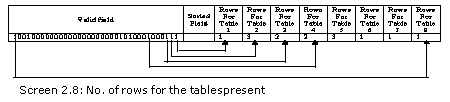The bytes following the sorted field are a series of ints, which contain the number of rows enclosed in every table that subsists. So, if there exist 8 tables, then 8 values are placed at the end of the header. Every value relates to a corresponding table present in the file.

Thus, the tableoffset variable points to the first int, which contains the number of rows in the first table type. The second int in sequence contains the count of rows for the second table type, and so on. Thus, with every new bit that is switched on, there exists an int that signifies the row count. An integer occupies 4 bytes. Hence, the value of tableoffset increases by 4 for every int that is added.

Thus, at each instance, k refers to the bit index and tableoffset points to the rows in the table. The static function Clear, of the array class, clears the array name from the specified location. The first parameter to the function is the arrayname. The second parameter is the starting point or the index. The third parameter is the ending index, which contains the number of bytes that are to be cleared. Since we want the entire array to be cleared, an offset of zero is specified, with the array length as the last parameter.

Thus, when the loop concludes, the rows array will contain a count of the rows for the tables that are present, and a value of zero for the tables that are absent.

Now, let us attempt and identify the tablenames for the tables present in the program.

Each table in the metadata world is assigned a number and a name. Thus, we create an array of strings, with these names mentioned at the right index position. The table with number 0 is known as Module, while the table with the number 1 is called TypeRef, and so on.

Now, using a 'for' loop that iterates 64 times, along with an 'if' statement, the tables types are displayed. This is carried out only for the tables that are present, or for those with a row count of one or more. The table name is displayed by reading the corresponding index off the tablenames array.

Finally, the value stored in the variable tableoffset is displayed. It points to the table data. Now, prior to forging ahead to the table data, let us display the #Strings stream.

a.cs

using System;

using System.IO;

public class zzz

{

public void DisplayGuid(int st)

{

Console.Write("{");

Console.Write("{0}{1}{2}{3}", guid[st+2].ToString("X") , guid[st+1].ToString("X") , guid[st].ToString("X") , guid[st-1].ToString("X"));

Console.Write("-{0}{1}-",guid[st+3].ToString("X") , guid[st+4].ToString("X"));

Console.Write("{0}{1}-",guid[st+6].ToString("X") , guid[st+5].ToString("X"));

Console.Write("{0}{1}-",guid[st+7].ToString("X") , guid[st+8].ToString("X"));

Console.Write("{0}{1}{2}{3}{4}{5}",

guid[st+9].ToString("X"),guid[st+10].ToString("X"),

guid[st+11].ToString("X"),guid[st+12].ToString("X")

,guid[st+13].ToString("X"),guid[st+14].ToString("X"));

Console.Write("}");

}

public string GetString(int starting)

{

int i = starting;

while (strings[i] != 0 )

{

i++;

}

System.Text.Encoding e = System.Text.Encoding.UTF8;

string  s = e.GetString(strings, starting , i - starting  );

return s;

}

public static void Main ()

{

zzz a = new zzz();

a.abc();

}

int tableoffset ;

int [] rows;

int [] offset;

int [] ssize ;

byte [] strings;

byte [] us;

byte [] guid;

byte [] blob;

long valid ;

byte [][] names;

public void abc()

{

FileStream s = new FileStream("C:\\mdata\\b.exe",FileMode.Open);

s.Seek(360, SeekOrigin.Begin);

int rva,size;

int where = rva%0x2000 + 512;

s.Seek(where + 4 + 4, SeekOrigin.Begin);

where = rva%0x2000 + 512;

s.Seek(where, SeekOrigin.Begin);

s.Seek(4 + 2 + 2 + 4 + 4 + 12 + 2, SeekOrigin.Current);

offset = new int;

ssize  = new int;

names = new byte[];

names = new byte;

names = new byte;

names = new byte;

names = new byte;

names = new byte;

int i = 0; int j ;

for ( i = 0 ; i < streams ; i++)

{

j = 0;

byte bb ;

while ( true )

{

if ( bb == 0)

break;

names[i][j] = bb;

j++;

}

names[i][j] = bb;

while ( true )

{

if ( s.Position % 4 == 0 )

break;

if ( b != 0)

{

s.Seek(-1, SeekOrigin.Current);

break;

}

}

}

for ( i = 0 ; i < streams ; i++)

{

if ( names[i] == '~' )

{

for ( int k = 0 ; k < ssize[i] ; k ++)

}

if ( names[i] == 'S' )

{

strings = new byte[ssize[i]];

for ( int k = 0 ; k < ssize[i] ; k ++)

}

if ( names[i] == 'U' )

{

us = new byte[ssize[i]];

for ( int k = 0 ; k < ssize[i] ; k ++)

}

if ( names[i] == 'G' )

{

guid = new byte[ssize[i]];

for ( int k = 0 ; k < ssize[i] ; k ++)

}

if ( names[i] == 'B' )

{

blob = new byte[ssize[i]];

for ( int k = 0 ; k < ssize[i] ; k ++)

}

}

tableoffset = 24;

rows = new int;

Array.Clear (rows, 0, rows.Length);

for ( int k = 0 ; k <= 63 ; k++)

{

int tablepresent = (int)(valid >> k ) & 1;

if ( tablepresent == 1)

{

tableoffset += 4;

}

}

xyz();

}

public void xyz()

{

for ( int k = 0 ; k < ssize ; k ++ )

{

Console.Write("{0}" , (char) strings[k]);

if ( strings[k] == 0 )

Console.WriteLine();

}

string s;

s = GetString(10);

Console.WriteLine("{0}" , s);

s = GetString(16);

Console.WriteLine("{0}" , s);

DisplayGuid(1);

}

}

Output

<Module>

b.exe

mscorlib

System

Object

zzz

Main

.ctor

System.Diagnostics

DebuggableAttribute

b

Console

WriteLine

b.exe

mscorlib

{A921C043-32F-4C5C-A5D8-C8C3986BD4EA}

This program displays the contents of the strings stream. A major slice of the code remains unchanged, except for the introduction of the function xyz. In brief, we have loaded the metadata information from the disk into five arrays. The rows array gives a count of the rows of each table. Then, we arrive at the tableoffset for the first table in the metadata array.

Now, let us explore the xyx function. This function will be encountered repeatedly in the future. Henceforth, we shall add all the new code in the xyz function only.

The strings stream is constituted of strings that are null terminated. So, in the 'for' loop, when a null or a zero is encountered, the program proceeds to a new line. This is achieved by utilizing the WriteLine function without any parameters, off the Console class.

Simultaneously, there is a function named GetString, which returns a string when it is passed a number or offset in the strings stream array. This can be achieved by using the GetString function in the Encoding class, which accepts 3 parameters, i.e. a byte array, the start position and the end position. It then returns a string.

The start position is easily available, as it is provided as a parameter to the GetString function code. However, to retrieve the end position, a 'while' loop is implemented, which quits on coming across a 0. In the loop, a variable i is initialized to the start position and thereafter, it is incremented constantly. When the loop quits, i contains the end position of the string. The values are supplied to the GetString function in the Encoding class, which stores the return value in the string variable s. The GetString function has been put to  extensive use in all the chapters.

There is one last function called DisplayGuid. This function contains only Write functions. A Globally Unique Identifier (GUID) consists of 16 bytes, i.e. 128 bits. This 128-bit number is unique across time and space.

Thus, whenever an entity has to be uniquely identified, it is assigned a GUID. There is an RFC on the Internet that has the algorithm to compute the GUID. In the DisplayGuid method, we have merely displayed the GUID by interchanging certain bytes, which is the manner in which the rest of the world displays them. We have read the GUID stream into the guid array, lest you have forgotten.